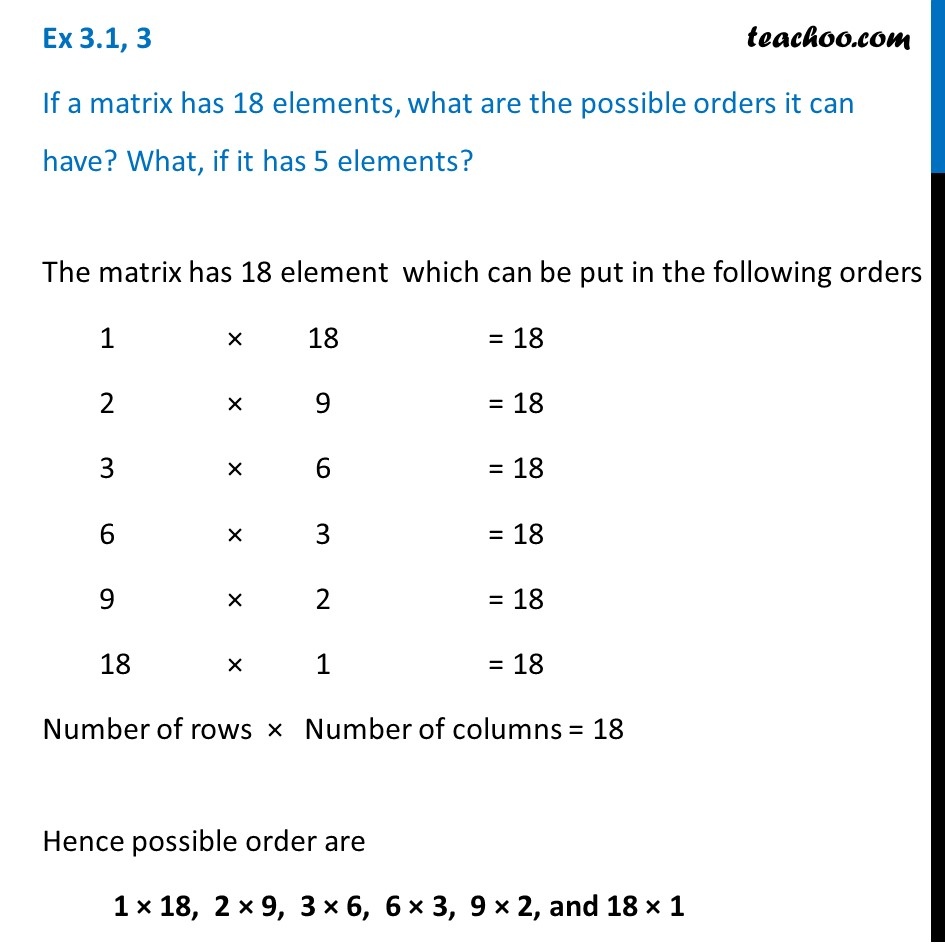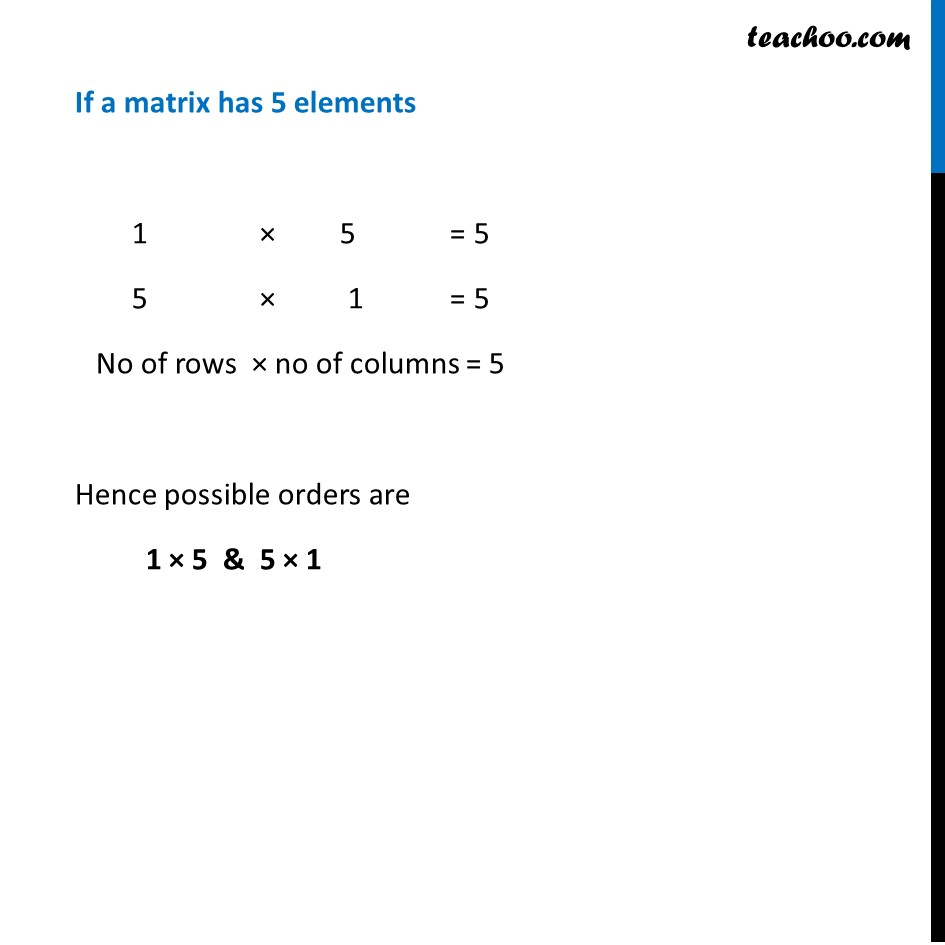Ex 3.1

Chapter 3 Class 12 Matrices
Serial order wiseLearn in your speed, with individual attention - Teachoo Maths 1-on-1 Class

### Transcript

Ex 3.1, 3 If a matrix has 18 elements, what are the possible orders it can have? What, if it has 5 elements? The matrix has 18 element which can be put in the following orders 1 × 18 = 18 2 × 9 = 18 3 × 6 = 18 6 × 3 = 18 9 × 2 = 18 18 × 1 = 18 Number of rows × Number of columns = 18 Hence possible order are 1 × 18, 2 × 9, 3 × 6, 6 × 3, 9 × 2, and 18 × 1 If a matrix has 5 elements 1 × 5 = 5 5 × 1 = 5 No of rows × no of columns = 5 Hence possible orders are 1 × 5 & 5 × 1